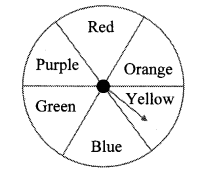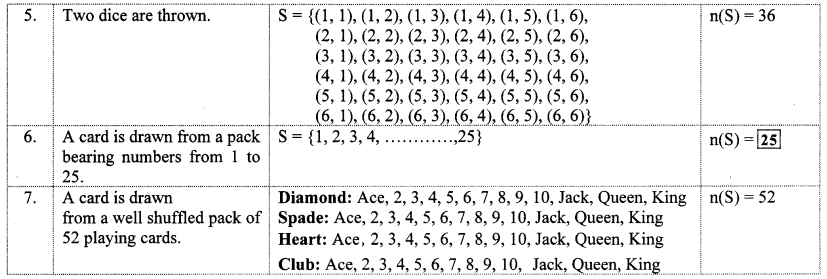# Maharashtra Board 10th Class Maths Part 1 Practice Set 5.2 Solutions Chapter 5 Probability

Balbharti Maharashtra State Board Class 10 Maths Solutions covers the Practice Set 5.2 Algebra 10th Class Maths Part 1 Answers Solutions Chapter 5 Probability.

## Practice Set 5.2 Algebra 10th Std Maths Part 1 Answers Chapter 5 Probability

Question 1.
For each of the following experiments write sample space ‘S’ and number of sample Point n(S)
i. One coin and one die are thrown simultaneously.
ii. Two digit numbers are formed using digits 2,3 and 5 without repeating a digit.
Solution:
i. Sample space,
S = {(H, 1), (H, 2), (H, 3), (H, 4), (H, 5), (H, 6), (T, 1), (T, 2), (T, 3), (T, 4), (T, 5), (T, 6)}
∴ n(S) =12
ii. Sample space,
S = {23,25,32, 35, 52, 53}
∴ n(S) = 6

Question 2.
The arrow is rotated and it stops randomly on the disc. Find out on which colour it may stop.Solution:
There are total six colours on the disc.
Sample space,
S = {Red, Orange, Yellow, Blue, Green, Purple}
∴ n(S) = 6
∴ Arrow may stop on any one of the six colours.

Question 3.
In the month of March 2019, find the days on which the date is a multiple of 5. (see the given page of the calendar).Solution:
Dates which are multiple of 5:
5,10, 15,20,25,30
∴ S = {Tuesday, Sunday, Friday, Wednesday, Monday, Saturday}
∴ n(S) = 6
∴ The days on which the date will be a multiple of 5 are Tuesday, Sunday, Friday, Wednesday, Monday and Saturday.

Question 4.
Form a ‘Road safety committee’ of two, from 2 boys (B1 B2) and 2 girls (G1, G2). Complete the following activity to write the sample space.
Solution:Question 1.
Sample Space

• The set of all possible outcomes of a random experiment is called sample space.
• It is denoted by ‘S’ or ‘Ω’ (omega).
• Each element of a sample space is called a sample point.
• The number of elements in the set S is denoted by n(S).
• If n(S) is finite, then the sample space is called a finite sample space.

Some examples of finite sample space. (Textbook pg. no, 117)
Solution:Scroll to Top